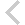FICO Xpress Optimization Examples Repository
 FICO Optimization Community FICO Xpress Optimization HomeIntroductory examples

Description
Introductory examples from the whitepaper 'Robust Optimization with Xpress'. Topics covered by the examples:
• Price of robustness: cost_of_robustness.mos (calculating the cost of having uncertainty in the model)
• Working with nominal values:

nominalvalue_0base.mos (simple robust model without nominal values);

nominalvalue_2base.mos (simple robust model with a nominal valued uncertain);

nominalvalue_none.mos (uncertainty as an addition to a coefficient);

nominalvalue_rule2.mos (using uncertains with nominal value as coefficients);

nominalvalue_shift1.mos (basic version without nominal values);

nominalvalue_shift2.mos (defining nominal values);

nominalvalue_shift3.mos (substituting the effect of the nominal values);

nominalvalue_simple.mos (uncertainty as a coefficient)
• Overlapping uncertainty:

overlapping_cardinality.mos (cardinality constraint with overlapping uncertainty sets);

overlapping_polyhedral.mos, overlapping_polyhedral2.mos (polyhedral uncertainty set with overlapping use in robust constraints)
• Working with scenarios:

scenario_simple.mos (simple robust optimization model with scenario based uncertainty);

scenario_simple_deterministic.mos (deterministic version of the simple scenario problem)
• Special cases:

careful_equalities.mos (robust problem with equality constraint);

careful_unbounded_uncertain.mos (robust problem with an unbounded uncertain)
Further explanation of this example: Whitepaper 'Robust Optimization with Xpress', Section 1 Introduction

Source Files

cost_of_robustness.mos

(!******************************************************
Mosel Example Problems
======================

Example model for the
Robust Optimization with Xpress white paper

(c) 2014 Fair Isaac Corporation

*******************************************************!)
model PriceRobustness
uses "mmrobust"                    ! Load the robust library

declarations
x : mpvar
u : uncertain
end-declarations

0 <= u
u <= 3

(1+u)*x <= 1

maximize(XPRS_NOMINAL, x)
nominal_objective := getobjval
writeln("Objective at nominal values:", nominal_objective );

maximize(x)
robust_objective := getobjval
writeln("Robust objective:", robust_objective );
writeln("Price of robustness:", nominal_objective - robust_objective );
writeln("Worst value of uncertain:", u.sol)

end-model